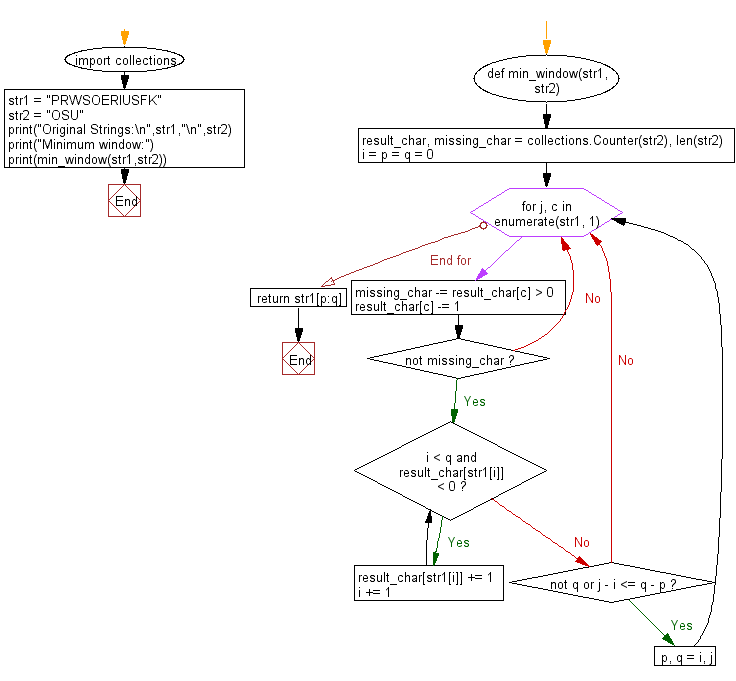﻿ Python: Find the minimum window in a given string which will contain all the characters of another given string - w3resource# Python: Find the minimum window in a given string which will contain all the characters of another given string

## Python String: Exercise-74 with Solution

Write a Python program to find the minimum window in a given string which will contain all the characters of another given string.

Example 1
Input
str1 = " PRWSOERIUSFK "
str2 = " OSU "
Output:
Minimum window is "OERIUS"

Sample Solution:

Python Code:

``````import collections
def min_window(str1, str2):
result_char, missing_char = collections.Counter(str2), len(str2)
i = p = q = 0
for j, c in enumerate(str1, 1):
missing_char -= result_char[c] > 0
result_char[c] -= 1
if not missing_char:
while i < q and result_char[str1[i]] < 0:
result_char[str1[i]] += 1
i += 1
if not q or j - i <= q - p:
p, q = i, j
return str1[p:q]

str1 = "PRWSOERIUSFK"
str2 = "OSU"
print("Original Strings:\n",str1,"\n",str2)
print("Minimum window:")
print(min_window(str1,str2))
```
```

Sample Output:

```Original Strings:
PRWSOERIUSFK
OSU
Minimum window:
OERIUS
```

Pictorial Presentation:Flowchart:## Visualize Python code execution:

The following tool visualize what the computer is doing step-by-step as it executes the said program:

Python Code Editor:

Have another way to solve this solution? Contribute your code (and comments) through Disqus.

What is the difficulty level of this exercise?

Test your Programming skills with w3resource's quiz.

﻿

## Python: Tips of the Day

Check if a given key already exists in a dictionary:

In is the intended way to test for the existence of a key in a dict.

```d = {"key1": 10, "key2": 23}

if "key1" in d:
print("this will execute")

if "nonexistent key" in d:
print("this will not")
```

If you wanted a default, you can always use dict.get():

```d = dict()

for i in range(100):
key = i % 10
d[key] = d.get(key, 0) + 1
```

and if you wanted to always ensure a default value for any key you can either use dict.setdefault() repeatedly or defaultdict from the collections module, like so:

```from collections import defaultdict

d = defaultdict(int)

for i in range(100):
d[i % 10] += 1
```

but in general, the in keyword is the best way to do it.

Ref: https://bit.ly/2XPMRyz